Homework Help Question & Answers

# Review | Constants l Periodic Table Complete the following table for an ideal gas: 2.20 atm...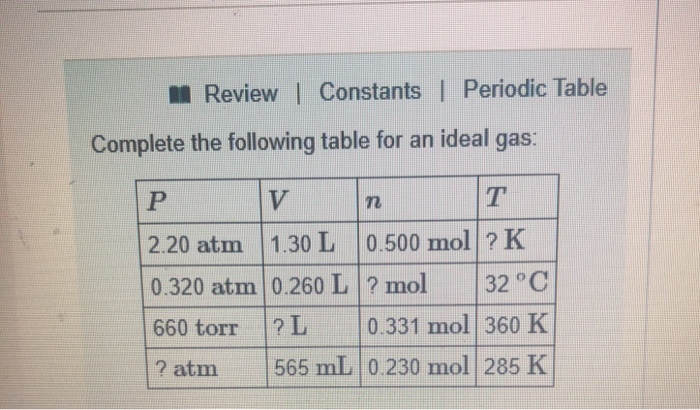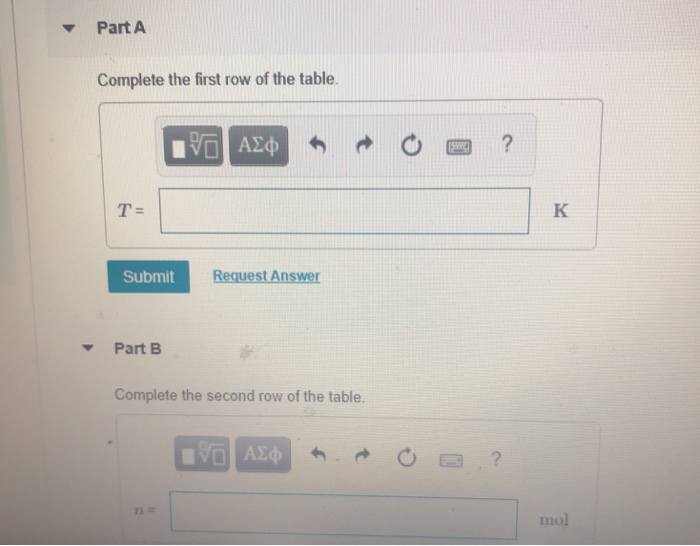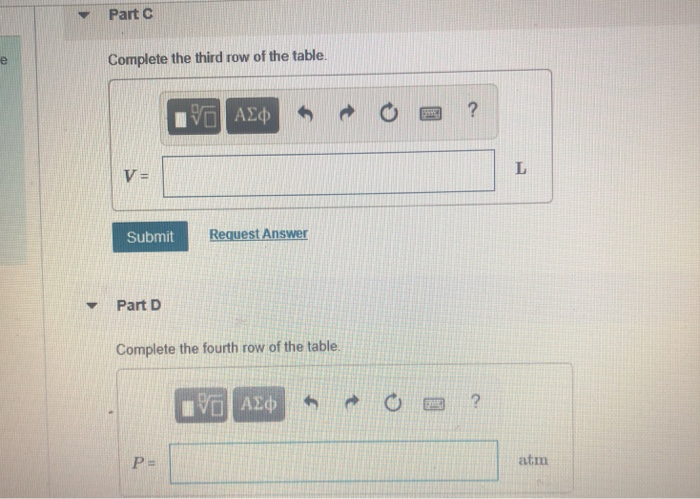Review | Constants l Periodic Table Complete the following table for an ideal gas: 2.20 atm | 1.30 L | 0.500 mol ? K 0.320 atm 0.260 L ? mol32°C 660 torr ?L 0.331 mol 360 K ? atm 565 mL 0.230 mol 285 K
Part A Complete the first row of the table. Request Answer Submut Part B Complete the second row of the table. mol
▼ Part C Complete the third row of the table. Request Answer Submit Y Part D Complete the fourth row of the table. atm

#### Homework Answers

Answer #1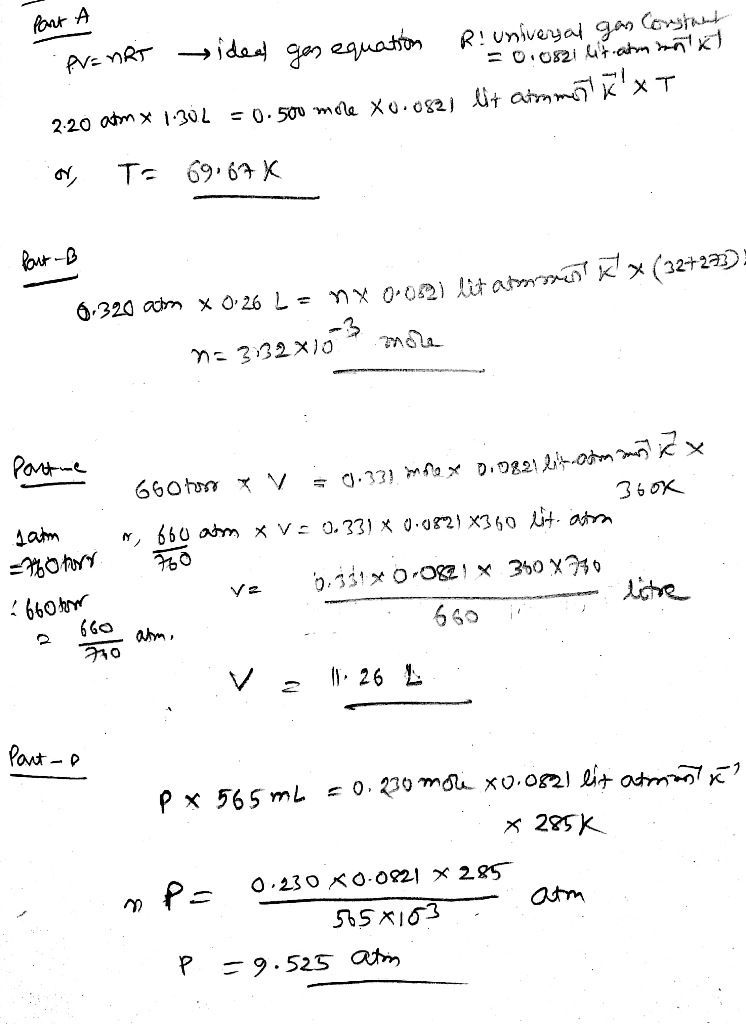Know the answer?
Your Answer:

#### Post as a guest

Your Name:

What's your source?

#### Earn Coin

Coins can be redeemed for fabulous gifts.

Not the answer you're looking for? Ask your own homework help question. Our experts will answer your question WITHIN MINUTES for Free.
Similar Homework Help Questions
• ### can anybody help me with this ? CH 5 Problem 10.33- Enhanced -with Feedback may want...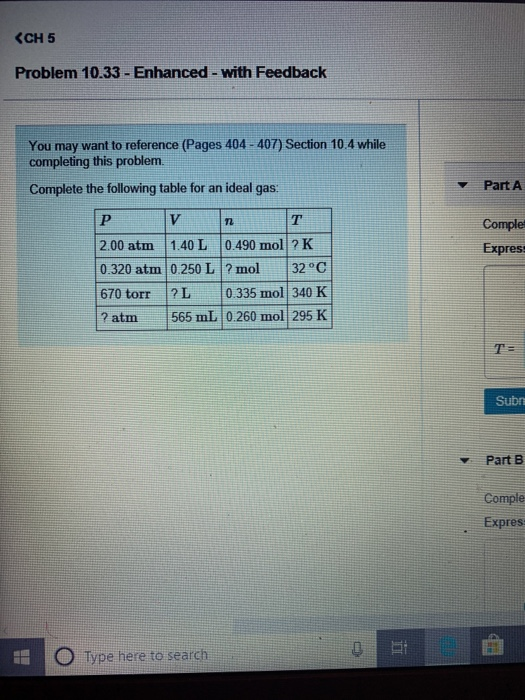can anybody help me with this ? CH 5 Problem 10.33- Enhanced -with Feedback may want to reference (Pages 404-407) Section 10.4 while completing this problem. You Part A Complete the following table for an ideal gas: T V P Comple 0.490 mol ? K 2.00 atm 1.40 L Expres 32°C 0.320 atm 0.250 L ? mol ? L 0.335 mol 340 K 670 torr 565 mL 0.260 mol 295 K ? atm T Subr Part B Comple Expres Type...

• ### 10-9 Review | Constants Periodic Table Calculate each of the following quantities for an ideal gas....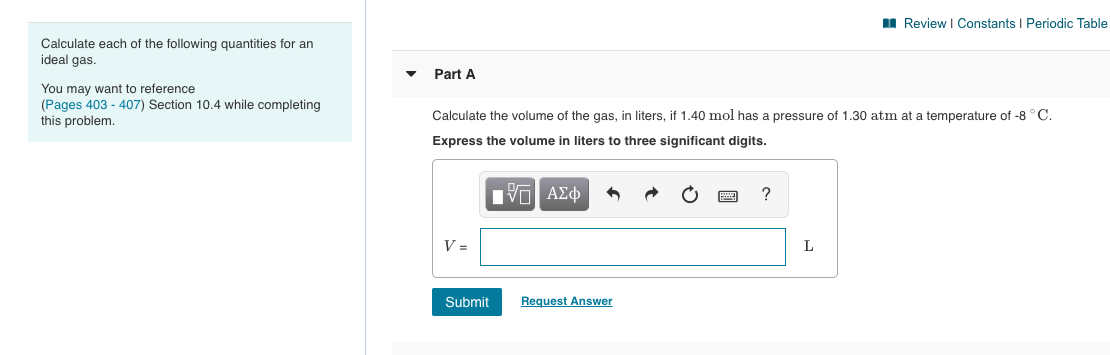10-9 Review | Constants Periodic Table Calculate each of the following quantities for an ideal gas. Part A You may want to reference (Pages 403 - 407) Section 10.4 while completing this problem. Calculate the volume of the gas, in liters, if 1.40 mol has a pressure of 1.30 atm at a temperature of -8°C. Express the volume in liters to three significant digits. O ACQ* * O O ? Submit Request Answer Part B Calculate the absolute temperature of...

• ### 10.33 Complete the following table for an ideal gas 10.33 Complete the following table for an...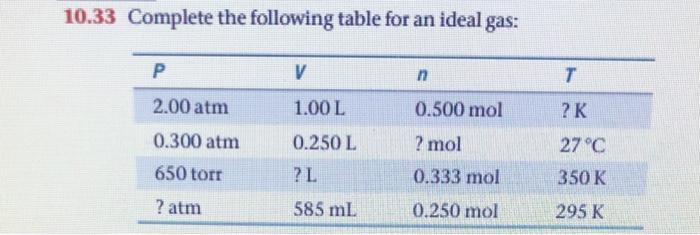10.33 Complete the following table for an ideal gas 10.33 Complete the following table for an ideal gas: P V n 1.00L 0.500 mol ?K 2.00 atm 0.300 atm 650 torr ? mol 27°C 0.250 L ? L 0.333 mol 350 K ? atm 585 ml 0.250 mol 295 K

• ### ㎜ Review Constants Periodic Table Part A gas has a Henry's law constant of 0.183 M/atm...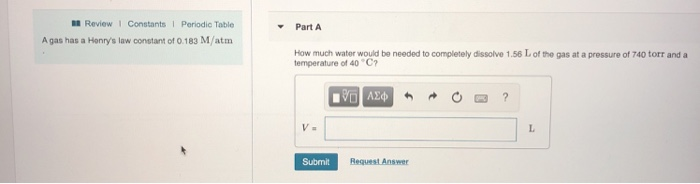㎜ Review Constants Periodic Table Part A gas has a Henry's law constant of 0.183 M/atm How much water would be needed to completely dissolve 1.56 L of the gas at a pressure of 740 torr and a temperature ot 40 C Submit Request Answer

• ### 2 Review Constants Periodic Table Complete the following table: Part A H01 (OH) pH POH Acidio...2 Review Constants Periodic Table Complete the following table: Part A H01 (OH) pH POH Acidio Basic or Neutral 7.3 x 10-M Complete the first column Complete the first column from top to bottom. Express your answers using two significant figures separated by commas. 1.99 5.6 x 10-5M V AEO ? 2.91 M Submit Request Answer Complete the following table: Part B H01 [OH-] pH POH Acidic, Basic or Neutral ? Complete the second column Complete the second column from...

• ### ent ( 3 of 10 Review | Constants Periodic Table Part A mixture initially contains A,...ent ( 3 of 10 Review | Constants Periodic Table Part A mixture initially contains A, B, and in the following concentrations: [A] -0.400 M B - 1.40 M and C -0.650 M. The following reaction occurs and equilibrium is established: A + 2B=C At equilibrium, (A) - 0.260 Mand (C -0.790 M. Calculate the value of the equilibrium constant, K. Express your answer numerically. View Available Hint(s) AEO K = Submit Show All X Review I Constants Periodic Table...

• ### hapter 15 Question 21- Algorithmic 9 of 18 > l Review I Constants| Periodic Table ▼...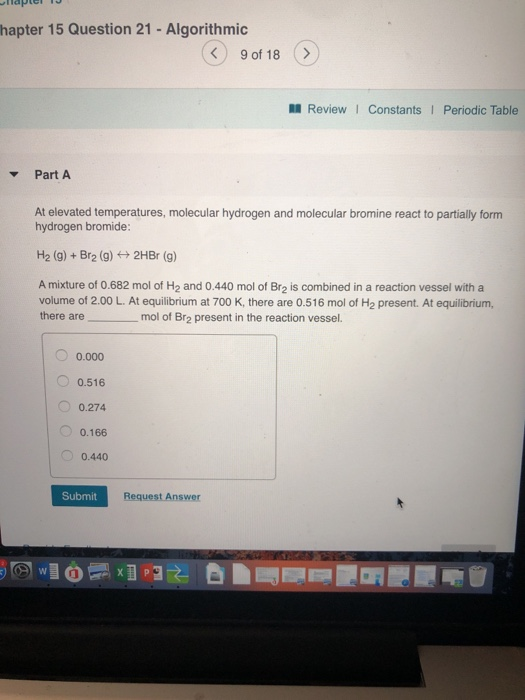hapter 15 Question 21- Algorithmic 9 of 18 > l Review I Constants| Periodic Table ▼ Part A At elevated temperatures, molecular hydrogen and molecular bromine react to partially form hydrogen bromide: H2 (g)+Br2 (g)2HBr (g) A mixture of 0.682 mol of H2 and 0.440 mol of Br2 is combined in a reaction vessel with a volume of 2.00 L. At equilibrium at 700 K, there are 0.516 mol of H2 present. At equilibrium, there are mol of Br2 present...

• ### ㎜ Review 1 Constants l Periodic Table Part A Consider the following reaction: H2 (g) +...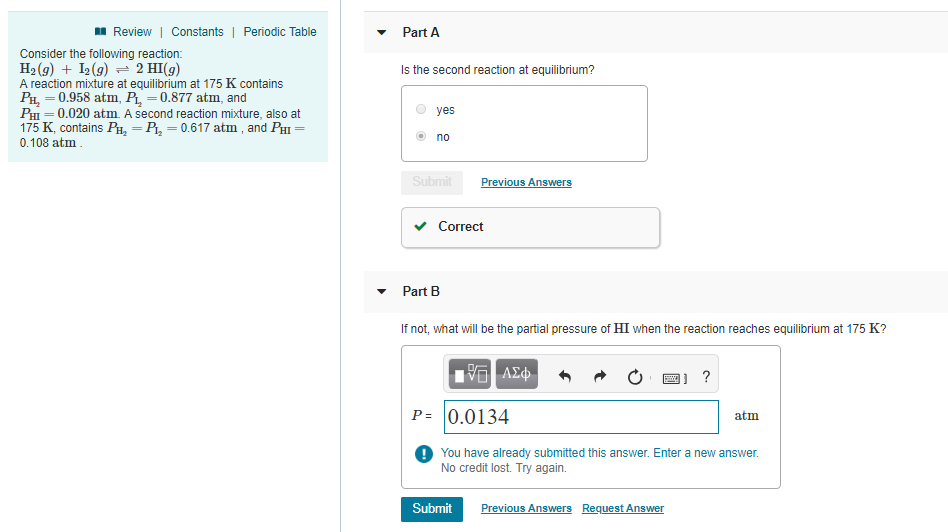㎜ Review 1 Constants l Periodic Table Part A Consider the following reaction: H2 (g) + 12(g) 2 HI(g) A reaction mixture at equilibrium at 175 K contains PH,0.958 atm, PL,0.877 atm, and PHI = 0.020 atm. A second reaction mixture, also at 175 K, contains PH, -P,-0.617 atm, and PHI 0.108 atm Is the second reaction at equilibrium? yes no revious Ans Correct Part B If not, what will be the partial pressure of HI when the reaction reaches...

• ### Complete the table for an ideal gas. Reaction Pressure Volume n T 1 1.5 atm 1.5...

Complete the table for an ideal gas. Reaction Pressure Volume n T 1 1.5 atm 1.5 L 0.100 moles [answer1] 2 780 torr 250 mL [answer2] 25°C 3 1.0 atm [answer3] 0.500 moles 300 K

• ### Q6 ( 6 of 10 Review | Constants Periodic Table Part A If the total volume...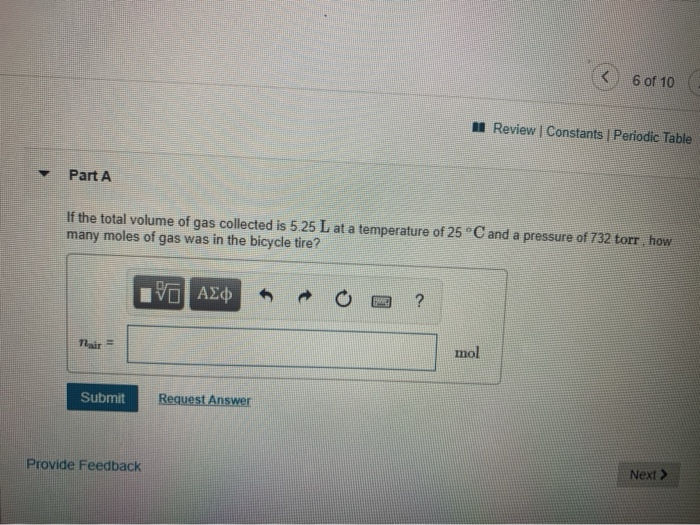Q6 ( 6 of 10 Review | Constants Periodic Table Part A If the total volume of gas collected is 5.25 L at a temperature of 25 °C and a pressure of 732 torr, how many moles of gas was in the bicycle tire? 10 AZ OO ? mol mol Submit Request Answer Provide Feedback Next > Item 6 The air in a bicycle tire is bubbled through water and collected at 25°C.

Free Homework App

Scan Your Homework
to Get Instant Free Answers
Need Online Homework Help?

Get Answers For Free
Most questions answered within 3 hours.